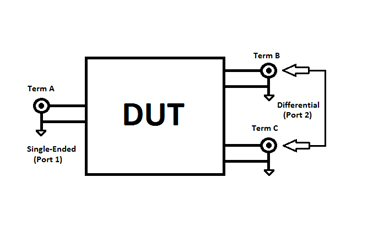# Mixed-Mode S-Parameters

New for May 2014! Contributed by Bryant.

Mixed-mode S-parameters are used for the analysis of differential circuits, and provide the capability of analyzing the signal flow through differential/balanced lines. S-parameters were originally developed to analyze single-ended/unbalanced networks. But why limit ourselves to describing single-ended networks? We can adapt these single-ended S-parameters to describe differential networks to report differential and common modes of operation which are commonly known as mixed-mode S-parameters[ref1, ref2]. The following paragraphs will describe the conversion of single-ended S-parameters to mixed-mode S-parameters and uses a 3-port network as an example.Let’s imagine some 3-terminal differential device to be measured on a VNA like the one shown in figure 1. Terminal A represents the single-ended/unbalanced port and terminals B and C represent the differential/balanced port. When the DUT is tested on the Vector Network Analyzer (VNA), it is measured in a 50 ohm environment, and typically recorded in some form or another where the single-ended data is reported. This single-ended data can then be manipulated mathematically to report the mixed-mode scattering parameters. The nomenclature used to represent the three different modes is represented by d – differential, c – common, and s – single-ended. We can then use mixed-mode S-parameters to determine the single-ended/unbalanced input return loss, differential/balanced output return loss, differential-mode insertion loss, common-mode rejection ratio (CMRR), magnitude imbalance, and phase imbalance. The input reflection coefficient on the single-ended/unbalanced port is represented as S11ss and is equal to single-ended S11 results returned by the VNA. The differential/balanced output reflection coefficient can be represented as S22dd, and can be calculated using the single-ended S-parameter data using the equation below.

S22dd = 0.5 • [ S22ss - S23ss - S32ss + S33ss ]   (1)

The input and output VSWR can be determined from the reflection coefficients in the normal fashion and is covered in more detail on our VSWR page). Similarly, we can take -20 log of that linear magnitude to determine the return loss in decibels. Check out our return loss page here. The differential-mode insertion loss of the device can be represented as Sds21 and found from the single-ended S-parameters using the equation below.

S21ds = (1/√2) • [ S21ss - S31ss ]  (2)

Similarly the common-mode response can be derived from the single-ended S-parameters using the following equation.

S21cs = (1/√2) • [ S21ss + S31ss ]  (3)

The differential-mode and common-mode responses are typically expressed in decibels and can be found by taking 20 log of the linear magnitude value. The common-mode rejection ratio is a key figure of merit of any differential system because its primary role is to reject undesired common-mode currents with minimal impact on the desired differential-mode currents. It is defined as the ratio of powers of the differential gain to the common-mode gain found in equations (2) and (3).

CMRR = 20 • log[ S21ds / S21cs ]  (4)

The imbalance is also an important figure of merit when describing the performance of a differential circuit. As the name suggests, the imbalance is the measurable difference between the two single-ended balanced ports (Terminal B and Terminal C), and is typically reported in both magnitude and phase. At lower frequencies this inequality is typically negligible; however as the frequency is increased any offset in lead inductance will degrade the imbalance. The imbalance can be calculated using the single-ended S-parameters from the following equation.

Imbalance = - [ S21ss / S31ss ]  (5)

Author : Bryant